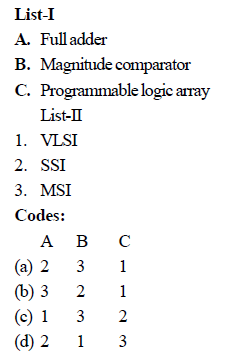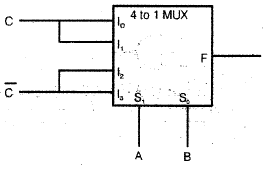# Test: Digital Electronics Circuits- 1

## 25 Questions MCQ Test Basic Electronics Engineering for SSC JE (Technical) | Test: Digital Electronics Circuits- 1

Description
Attempt Test: Digital Electronics Circuits- 1 | 25 questions in 100 minutes | Mock test for Electrical Engineering (EE) preparation | Free important questions MCQ to study Basic Electronics Engineering for SSC JE (Technical) for Electrical Engineering (EE) Exam | Download free PDF with solutions
QUESTION: 1

Solution:
QUESTION: 2

Solution:
QUESTION: 3

### The Boolean theorem AB + AC+ BC= AB + AC corresponds to

Solution:
QUESTION: 4

Match List- (Circuits) with List-II (Types of integration level) and select the correct answer using the codes given below the lists:Solution:
QUESTION: 5

Y = f(A,B) = IIM (0, 1, 2, 3) represents (M is Maxterm)

Solution:
QUESTION: 6

Consider the following statements regarding ICs:

1. ECL has the least propagation delay

.2. TTL has the largest fanout.

3. CMOS has the biggest noise margin.

4. TTL has the lowest power consumption.

Solution:
QUESTION: 7

For a logic family

VOH is the minimum output high level voltage

VOH is the maximum output low level voltage

VIH is the minimum acceptable input high level voltage

VIL is the maximm acceptable input low level voltage

The correct relationship among these is

Solution:
QUESTION: 8

The logic circuit realied by the circuit shown int the given figure will beSolution:
*Multiple options can be correct
QUESTION: 9

In a negative edge triggered J-K flip-flop, in order to have the output Q state 0, 0 and1 in the next three successive clock pulses, the J-K input states required would be respectively

Solution:
QUESTION: 10

The initial state of MOD- 16 down counter is 0110. After 37 clock pulses, the state of the counter will be

Solution:
QUESTION: 11

The minimum decimal equivalent of the number 11C.0 is

Solution:
QUESTION: 12

(FE35)16 XOR(CB15)16 is equal to

Solution:
QUESTION: 13

Which one of the following figures represents the  coincidence logic?

Solution:
QUESTION: 14

The figure of merit of a logic family is given by

Solution:
QUESTION: 15

Which one of the following statements correctly defines th full-adder? An adder circuit

Solution:
QUESTION: 16

The output of a JK flipflop with asynchronous preset and clear inputs is ‘1’. The output can be changed to ‘0’ with one of the following conditions

Solution:

Preset state of JK Flip-Flop =1 With J=1 K=1 and the clock next state will be complement of the present state

QUESTION: 17

Which one of the following can be used as parallel to series converter?

Solution:
QUESTION: 18

Consider the following statements:

A multiplexer

1. Selects on e of the several inputs and transmits it to a single output

2. routes the data from a single input to one of many output

3. converts parallel data into serial data

4. is a combinational circuit

Which of these statements are correct?

Solution:
QUESTION: 19

Consider the following statements:

Solution:
QUESTION: 20

A ring counter consisting of five flip-flops will have

Solution:
QUESTION: 21

A crystal oscillator is frequently used in digital circuits for timing purposes because of its

Solution:
QUESTION: 22

Which one of the following statement is correct?

Solution:
QUESTION: 23

F's complement of (2BFD)hex is

Solution:
QUESTION: 24

The number of digit 1 present in the binary representation of 3 × 512 + 7 × 64 + 5 × 8 + 3

Solution:
QUESTION: 25

If the output of a logic gate is '1' when all its inputs are at logic '0', the gate is either

Solution:Use Code STAYHOME200 and get INR 200 additional OFF Use Coupon Code